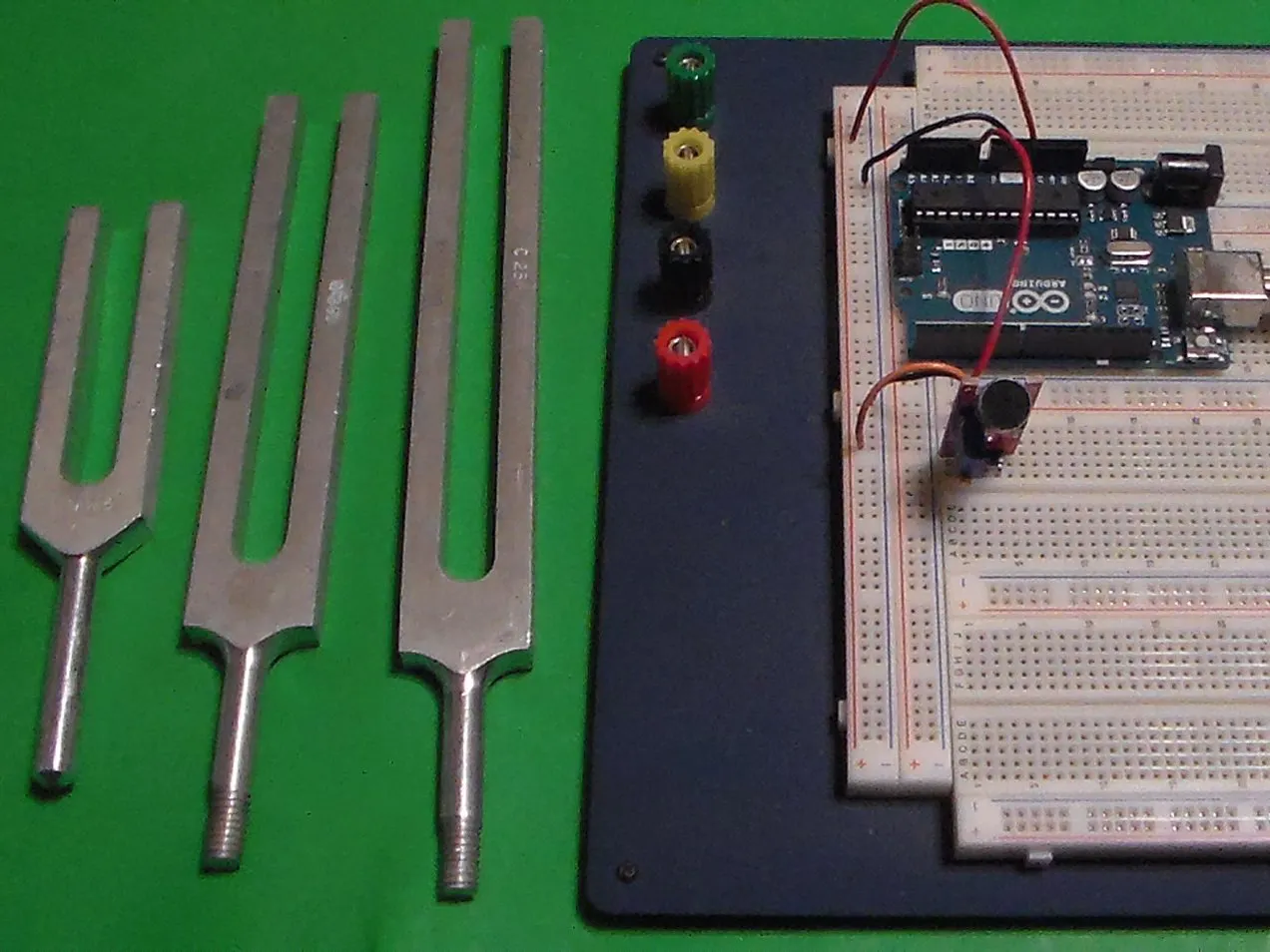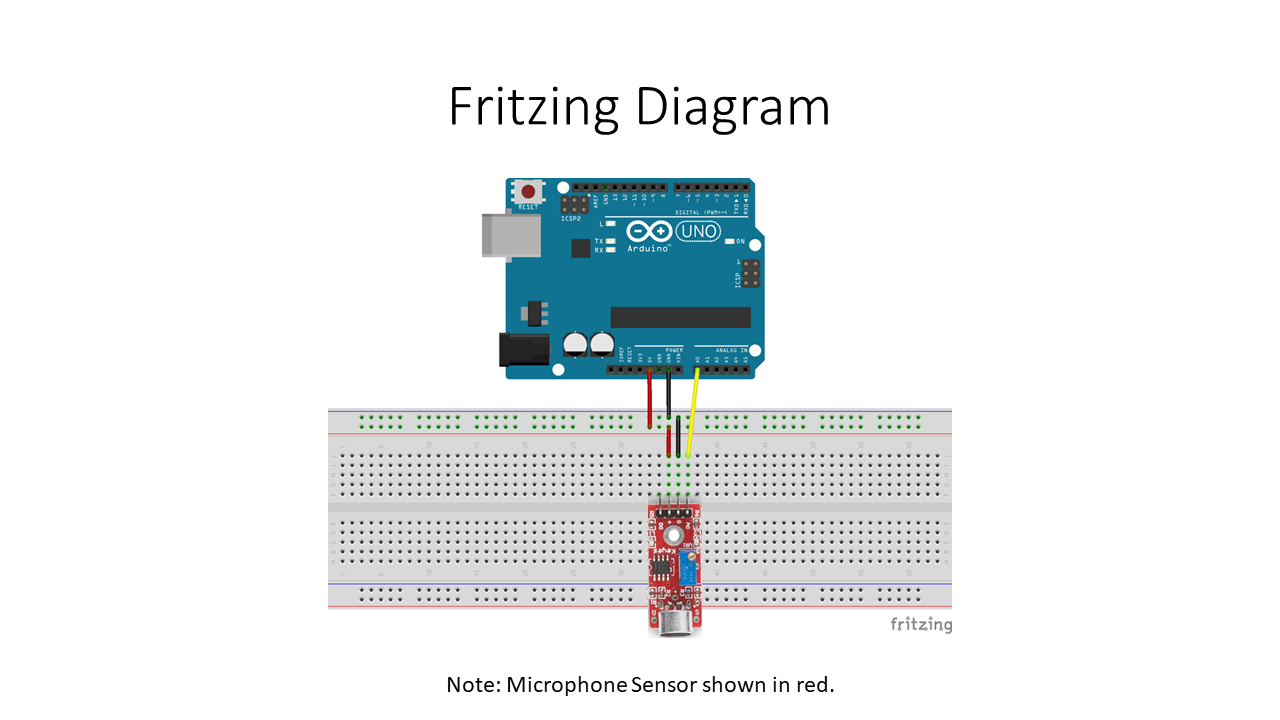Project tutorial# Audio Frequency Detector © GPL3+

This Arduino project displays the approximate frequency of the loudest sound detected by a sound detection module.

• 2,740 views
• 1 comment
• 10 respects

## Components and supplies

This Arduino project displays the approximate frequency of the loudest sound detected by a sound detection module. For this project, the analog output from the sound module detector sends the analog audio signal detected to A0 of the Arduino Uno. The analog signal is sampled and quantized (digitized). A Fast Fourier Transform (FFT) is then performed on the digitized data. The FFT converts the digital data from the approximate discrete-time domain result. The maximum frequency of the approximate discrete-time domain result is then determined and displayed via the Arduino IDE Serial Monitor.

## Code

##### AudioFrequencyDetectorV1-0.inoArduino
This code/sketch makes displays the approximate frequency of the loudest sound detected by a sound detection module.
```/*
File/Sketch Name: AudioFrequencyDetector

Version No.: v1.0 Created 12 December, 2019

Original Author: Clyde A. Lettsome, PhD, PE, MEM

Description:  This code/sketch makes displays the approximate frequency of the loudest sound detected by a sound detection module. For this project, the analog output from the
sound module detector sends the analog audio signal detected to A0 of the Arduino Uno. The analog signal is sampled and quantized (digitized). A Fast Fourier Transform (FFT) is
then performed on the digitized data. The FFT converts the digital data from the approximate discrete-time domain result. The maximum frequency of the approximate discrete-time
domain result is then determined and displayed via the Arduino IDE Serial Monitor.

Note: The arduinoFFT.h library needs to be added to the Arduino IDE before compiling and uploading this script/sketch to an Arduino.

License: This program is free software; you can redistribute it and/or modify it under the terms of the GNU General Public License (GPL) version 3, or any later

Notes: Copyright (c) 2019 by C. A. Lettsome Services, LLC

*/

#include "arduinoFFT.h"

#define SAMPLES 128             //SAMPLES-pt FFT. Must be a base 2 number. Max 128 for Arduino Uno.
#define SAMPLING_FREQUENCY 2048 //Ts = Based on Nyquist, must be 2 times the highest expected frequency.

arduinoFFT FFT = arduinoFFT();

unsigned int samplingPeriod;
unsigned long microSeconds;

double vReal[SAMPLES]; //create vector of size SAMPLES to hold real values
double vImag[SAMPLES]; //create vector of size SAMPLES to hold imaginary values

void setup()
{
Serial.begin(115200); //Baud rate for the Serial Monitor
samplingPeriod = round(1000000*(1.0/SAMPLING_FREQUENCY)); //Period in microseconds
}

void loop()
{
/*Sample SAMPLES times*/
for(int i=0; i<SAMPLES; i++)
{
microSeconds = micros();    //Returns the number of microseconds since the Arduino board began running the current script.

vReal[i] = analogRead(0); //Reads the value from analog pin 0 (A0), quantize it and save it as a real term.
vImag[i] = 0; //Makes imaginary term 0 always

/*remaining wait time between samples if necessary*/
while(micros() < (microSeconds + samplingPeriod))
{
//do nothing
}
}

/*Perform FFT on samples*/
FFT.Windowing(vReal, SAMPLES, FFT_WIN_TYP_HAMMING, FFT_FORWARD);
FFT.Compute(vReal, vImag, SAMPLES, FFT_FORWARD);
FFT.ComplexToMagnitude(vReal, vImag, SAMPLES);

/*Find peak frequency and print peak*/
double peak = FFT.MajorPeak(vReal, SAMPLES, SAMPLING_FREQUENCY);
Serial.println(peak);     //Print out the most dominant frequency.

/*Script stops here. Hardware reset required.*/
while (1); //do one time
}
```

## Schematics

This Arduino project displays the approximate frequency of the loudest sound detected by a sound detection module.#### Published on

December 18, 2019

#### Members who respect this project

See similar projects
you might like

#### Metal Detector Using Frequency Counter and OLED Display

Project tutorial by Andrius Purr

• 3,531 views
• 21 respects

#### Android Frequency Counter

Project tutorial by Projecter

• 2,985 views
• 5 respects

#### Frequency Counter for Android

Project tutorial by moty

• 4,390 views
• 11 respects

#### A PM2.5 and PM 10 Detector design for Windows 10 UWP App

Project tutorial by Jiong Shi

• 10,641 views
• 24 respects

#### Christmas Presents Intruder Detector

Project tutorial by David Escobar

• 4,402 views